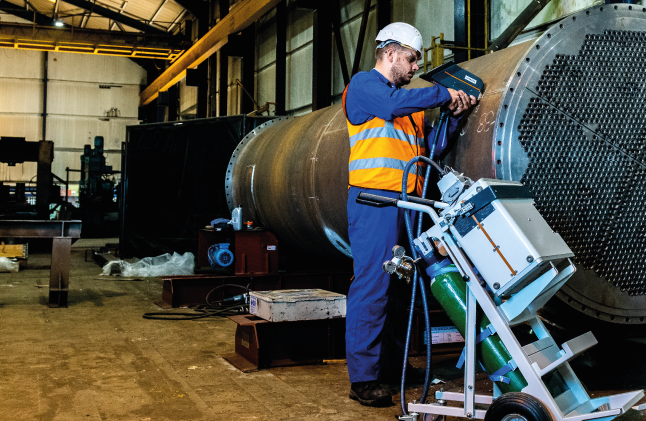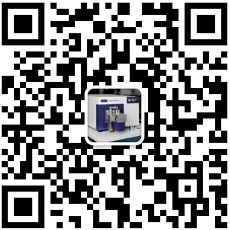﻿ PMI-MASTER Smart：更智能化的测试方法 日立便携式直读光谱仪-广州明阳机电有限公司

CE = (%C)+((%Mn)/6)+(((%Cr)+(%Mo)+(%V))/5)+(((%Cu)+(%Ni))/15)

CEM = (%C)+((%Si)/25)+(((%Mn)+(%Cu))/20)+(((%Cr)+(%V))/10)+((%Mo)/15)+((%Ni)/40)

CET = (%C)+(((%Mn)+(%Mo))/10)+(((%Cr)+(%Cu))/20)+((%Ni)/40)

CEV = (%C)+((%Mn)/6)+(((%Cu)+(%Ni))/15)+(((%Cr)+(%Mo)+(%V))/5)

PCM = (%C)+((%Si)/30)+(((%Cr)+(%Cu)+(%Cr))/20)+((%Ni)/60)+((%Mo)/15)+((%V)/10)+((%B)*5)

PMI-MASTER Smart是真正便携的高性能光谱仪，其重量仅为15 kg，体积小巧，可轻松携带至分析地点。它可以用一个电池进行多达300次分析，不需要预热。如果需要超低LOD的UVTouch激发枪，则需要20分钟的氩气吹扫时间。如果可接受200 ppm的LOD，则无需吹扫标准激发枪。

PMI-MASTER Smart凭借移动设备的便利性,在您使用火花直读光谱仪时,为您提供准确测量以及舒适体验。（本文摘至日立分析仪器微信公众号推文，用于技术交流，版权归作者所有，如有疑问请与我司联系）PMI-MASTER Smart：更智能化的测试方法 日立便携式直读光谱仪

>

PMI-MASTER Smart：更智能化的测试方法 日立便携式直读光谱仪